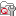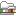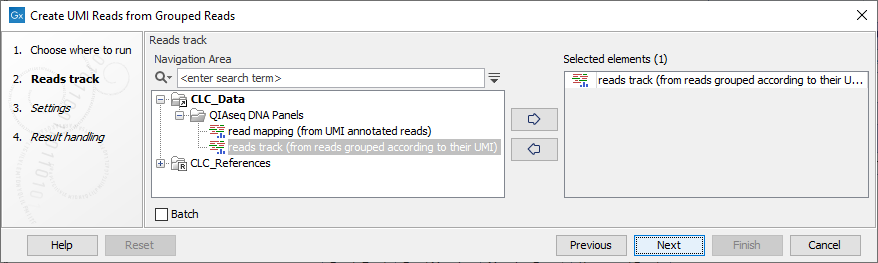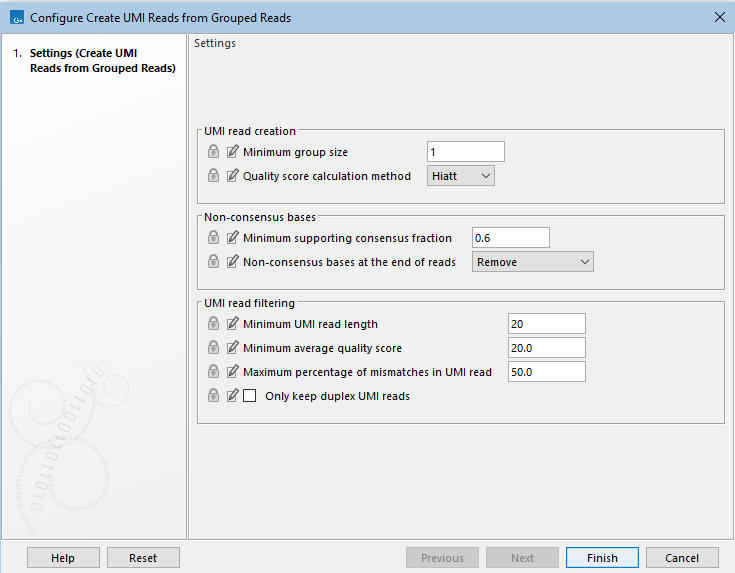The tool can be found in the Toolbox here:

Tools | QIAseq Panel Expert Tools () | QIAseq DNA Panel Expert Tools () | Create UMI Reads from Grouped Reads ()

In the first dialog (figure 5.39), select a read mapping of the original reads with UMI annotations that was previously handled with the Calculate Unique Molecular Index Groups tool.Figure 5.39: Select a read mapping of the original reads with UMI annotations.

The second dialog of the wizard (figure 5.40) offers the following options:Figure 5.40: Settings for the Create UMI Reads from Grouped Reads tool.

• Minimum group size: The tool will only create a UMI read if the number of reads in the UMI is at least "Minimum group size".

• Non-consensus bases
• Minimum supporting consensus fraction set at 0.6 by default. At each position in the UMI read, the consensus nucleotide is chosen to be the nucleotide with the highest probability of being correct (see the Consensus nucleotide calculation paragraph below). If this probability is higher than "Minimum supporting consensus fraction", a Q score for the consensus nucleotide is calculated. A position in UMI reads that does not have a consensus nucleotide will be considered a N with Q score 0.
• There is a choice between 3 methods of handling non-consensus bases (N with a Q score of 0) that are located at the end of the reads: Remove removes the bases, Keep as unaligned keeps the bases as unaligned ends, and Keep as aligned keeps the bases as aligned bases.

• Minimum UMI read length: UMI shorter than this value will be discarded.
• Minimum average quality score: UMI reads will be discarded, if their average Q-score is lower than "Minimum average quality score".
• Maximum percentage of mismatches in UMI read: UMI reads will be discarded, if more than this percentage of the bases are mismatches.

Click Next to Open or Save the resulting read mapping of UMI reads, i.e., a read mapping of the merged UMI groups. It is also possible to generate a report that will indicate how many reads were ignored and the reason why they were not included in a UMI read. This data will let you verify the found variants, and examine why expected variants were not found.

Consensus nucleotide calculation is performed following the method described in [Hiatt et al., 2013]. The consensus base is chosen so that the posterior probability of the observed read bases is maximized.

In order to maximize the posterior probability of calling a base, i.e.,where Oi is the observed base at a given position, C the base in question, and where all possible bases are summed up in the denominator, e.g. B=A,T,C,G.

Assuming that the prior for observing any base is equal, i.e., P(A)=P(T)=P(C)=P(G), then the posterior probability is:And by assuming each read base observation is independent,To obtain the consensus base we only need to maximize the numerator.

The Q-score is now simply the probability of making a wrong call, i.e.which means that the Q-score isQ-scores are capped at 60.